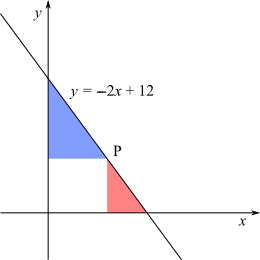#### You may also likeIn this problem we are faced with an apparently easy area problem, but it has gone horribly wrong! What happened?### Parabolic Patterns

The illustration shows the graphs of fifteen functions. Two of them have equations y=x^2 and y=-(x-4)^2. Find the equations of all the other graphs.### Exploring Cubic Functions

Quadratic graphs are very familiar, but what patterns can you explore with cubics?

# Graph Triangles

##### Age 14 to 16 Short Challenge Level:

This is the graph of the line $y=-2x+12$.Point P is on this line.

The ratio of the area of the red triangle to the area of the blue triangle is $1:4$.

Find the coordinates of point $\text P$.

This problem is adapted from the World Mathematics Championships
You can find more short problems, arranged by curriculum topic, in our short problems collection.